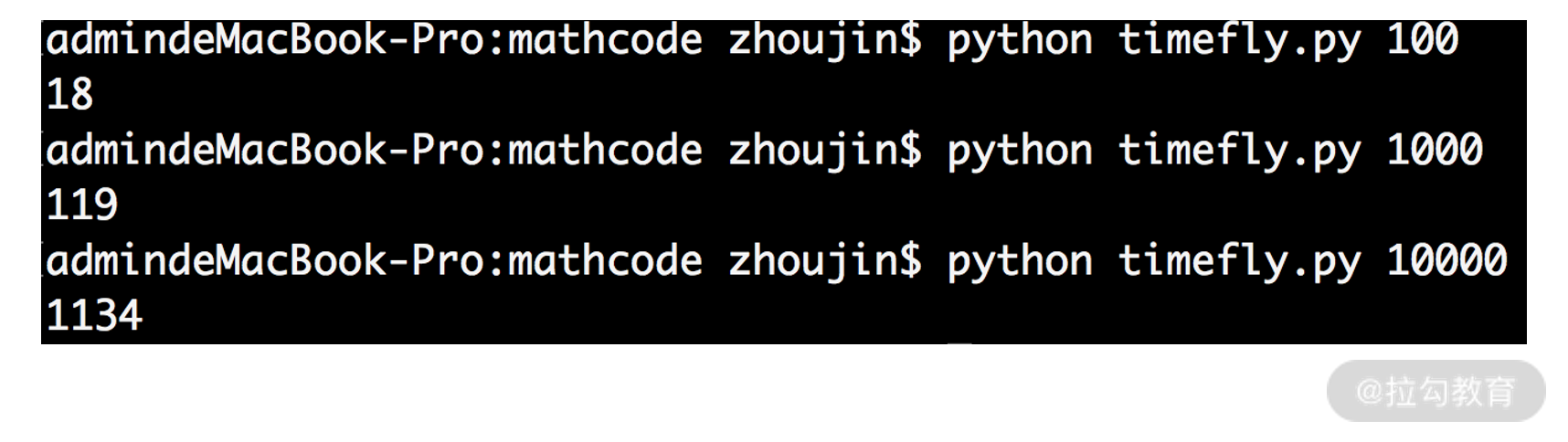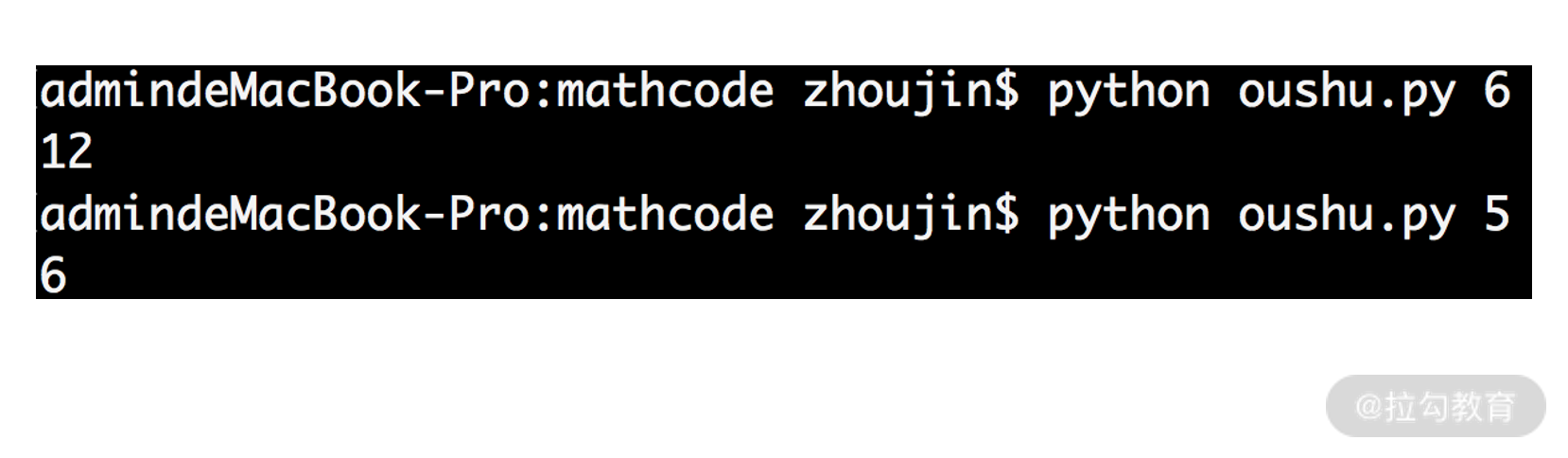13 复杂度：如何利用数学推导对程序进行优化？

程序的时间损耗

import time

import sys

t1 = int(time.time()*1000000)

n = int(sys.argv)

result = 0

for i in range(n):

result += i

t2 = int(time.time()*1000000)

print t2 - t1

• 第 4 行，记录了程序开始执行的毫秒级时间戳；
• 第 5 行，得到输入参数 n；
• 第 7～8 行，执行 1 加到 n 的循环求和；
• 第 9 行，记录了程序结束计算的毫秒级时间戳；
• 最后，第 10 行打印出程序执行的时间损耗。程序的复杂度

【例 1】我们先举一个例子说明，看下面这段代码：

a = [1,2,2,3,4,5]

result = 0

for i in range(len(a)):

result += a[i]

print result

【例 2】我们再看一个例子，代码如下：

a = [1,2,2,3,4,5]

result = 0

result = a + a[-1]

print result

复杂度的性质和代码结构

a = [1,2,2,3,4,5]

index_max = 0

times_max = -1

for i in range(len(a)):

times_temp = 0

for j in range(len(a)):

if a[i] == a[j]:

times_temp += 1

if times_temp > times_max:

times_max = times_temp

index_max = i

result = a[index_max]

for k in range(len(a)):

result += a[k]

print result

• 第 4～11 行，有两层 for 循环。我们具体算一下时间损耗，t(4～11行) = 6×[t(第4,5行)+t(6～8行)+t(9～11行)]。
• 而程序的第 6～8 行，又是一个 for 循环，则有 t(6～8行) = 6×t(第6,7,8行)
• 因此，整体的时间损耗为 t(4～11 行)= 6×[t(第4,5行) + 6×t(第6,7,8行)+ t(9～11行)] = n×n×b + n×c + n×d。

• 而第 14～15 行，根据前面所学是 O(n) 的时间复杂度。所以，整个代码的时间复杂度就是 O(n2+n)。仍然可以继续使用刚刚平方公式的化简方法，得到最终的时间复杂度是 O(n2)。

利用数学来优化时间复杂度

a = [2,1,4,3,4,2,3]

for i in range(0,len(a)):

times = 0

for j in range(0,len(a)):

if a[i] == a[j]:

times += 1

if times == 1:

print a[i]

break

• 第 2 行，开始 for 循环，并把计数的变量 times 置为 0；
• 第 4 行，嵌套了一个 for 循环；
• 第 5 行开始，判断里外两层循环的值是否相等。如果相等，则 times 加 1；
• 第 7 行，判断 times 是否为 1，如果为 1 说明 a[i] 在数组中只出现了一次，则打印并 break 跳出循环结束。

a = [2,1,4,3,4,2,3]

result = a

for i in range(1,len(a)):

result = result ^ a[i]

print result

• 第一，满足交换律和结合律；
• 第二，可以把相同元素计算为 0；
• 第三，0 异或任何数字都是其本身。

import sys

n = int(sys.argv)

result = 0

for i in range(n+1):

if i % 2 == 0:

result += i

print result

import sys

n = int(sys.argv)

a1 = 0

d = 2

nn = n/2 + 1

print nn * a1 + 2 * nn * (nn - 1) / d

• 第 2 行，获得输入变量 n。
• 第 3 行，求和的第一项，直接赋值为 0。
• 第 4 行，公差 d 为 2。
• 第 5 行，求项数。例如，输入 6，则项数为 0、2、4、6，6/3+1 = 4 项；输入 5，则项数为 0、2、4，5/2+1 = 3 项。
• 最后第 6 行，调用等差数列求和公式，直接得到结果，运行截图如下：